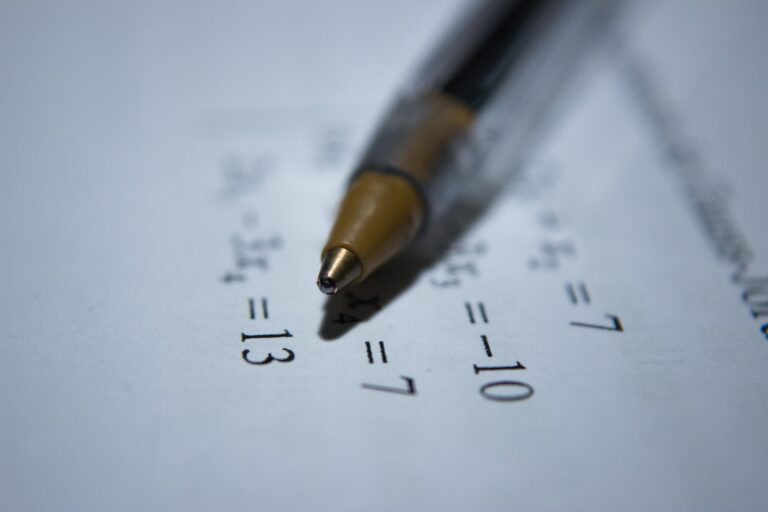# What Is Standard Form? Representation Of Different NumbersStandard form is commonly used in mathematics to represent a very large number in a small number in a particular manner. It is used to convert different numbers in a very common manner. It is used on a very large scale in the field of sciences and engineering. In mathematics, the standard form converts the equation into a canonical form and a generalized form of the numbers. Scientists are also used to measuring the magnitude of different quantities. Moreover, in the field of chemistry and physics makes calculation very easier for students.

In this article, we will discuss the definition of the standard form, formula, applies it to different types, and explain the complete idea with the help of examples.

## Definition of Standard Form

“The standard form is converting the larger number in smaller in the exponent of 10 and multiplying with the integer.”

The standard form is used to convert the number in the exponent of 10 and with the multiple of the integer.

The integer is greater or equal to 1 and must be less than 10. It may be positive or negative.

Formula:

B × 10n

Where B is the number such that 1≤ B < 10 and “n” is the integer (positive or negative number).

## The Standard Form of Numbers

In this section, we discuss the standard form of large numbers and how we can write them for different numbers.

To write the standard form steps are given below:

1. Write the first digit of the large number from the left side.

2. Add a decimal point after the first digit.

3. Write the remaining non-zero integer after the decimal point.

4. Count the number after the first digit and write in the form of an integer in the exponent of 10

## The Standard Form of Decimal Number

The decimal number is defined as the decimal point already placed in the number. (i.e. 66.67, 8273.3, 0.06732). The standard form these types of numbers is written as:

1. Firstly, write the first non-zero digit from the given number.

2. Place the decimal point after the first digit that writes first.

3. Write the remaining non-zero integer after the decimal point.

4. Count the number that is placed before the decimal point and it can write in the exponent of 10 as a positive integer. ( i.e., 66.67, 68.9367) If the number is in the form of (i.e., 0.0067, 0.00093702) then the 4th step skips and by following the above three steps similarly. Count the digits from the left side of the given number and write the exponent of the 10 as a negative integer.

### Examples

In this section, we discuss the examples of a standard form for its understanding.

#### Example 1

Find the standard form of the 6700007354.

Solution:

Step 1: Write the complete number.

6700007354

Step 2: write the first number.

6

Step 3: Add the decimal point after the first digit.

6.

Step 4: write the non-zero digit after the decimal point.

6. 7

Step 5: count the number and write the number in the exponent of 10.

6.7 × 109

6.7 × 109 is the standard form of 6700007354.

A standard form calculator can be used to convert the larger and smaller numbers in standard form along with engineering notation.

#### Example 2

Find the standard form of 9734000.

Solution:

Step 1: Write the complete number.

9734000

Step 2: write the first number.

9

Step 3: Add the decimal point after the first digit.

9.

Step 4: write the non-zero digit after the decimal point.

9. 734

Step 5: count the number and write the number in the exponent of 10.

9. 734 × 106

9. 734 × 106 is the standard form of 9734000.

#### Example 3

Find the standard form of 0.003354.

Solution:

Step 1: Write the complete number.

0.003354

Step 2: write the first non-zero number.

3

Step 3: Add the decimal point after the first digit.

3.

Step 4: write the non-zero digit after the decimal point.

3. 354

Step 5: count the number and write the number in the exponent of 10 in the form of a negative integer.

3. 354 × 10 -3

3. 354 × 10 -3 is the standard form of 0.003354.

#### Example 4

Find the standard form of the 8734.043.

Solution:

Step 1: Write the complete number.

8734.043

Step 2: write the first non-zero number.

8

Step 3: Add the decimal point after the first digit.

8.

Step 4: write the non-zero digit after the decimal point.

8. 734043

Step 5: count the number and write the number in the exponent of 10 in the form of a positive integer.

8. 734043 × 103

8. 734043 × 103 is the standard form of 8734.043.

## Summary

In this article, we have discussed the definition of standard form and a detailed discussion to write the decimal number in standard form. Moreover, for a better understanding of the standard form performed different examples.###### Carelle

Carelle is a teacher who has been through the ups and downs of the teacher and learner life. She wishes for every learner to gain educational satisfaction that will help embody the people they want to be in the future.

###### Tell Carelle Below What You Think About Her Post!

FamilyTutor is an established home tuition agency in Singapore! We match suitable home tutors for our clients not just to improve the students' academic grades, but also to build a strong rapport and meaningful relationship with the students and even the their whole family. FamilyTutor put every student in good hands!

If you need an excellent home tutor, feel free to call/WhatsApp us at +65 8777-2168! Our matching service is free!